# How to check if every segments of a road have the same directions?

2466
17
11-02-2017 03:35 AMby
New Contributor II

Hi,

I’m attempting to make a linear referencing. It’s a polyline M layer (with lengths along every line)  of forest roads . Every road (unique road number) consists of 1, 2 or more segments. In the attribute table I have a field with segment_id (unique in the layer) and a field with the road_number (many segments can have the same road_number). The layer is sorted ascending by segment_id and road_number.

The problem is – how to check if every segments of one road_number have the same direction. Maybe the solution is to calculate distance for end point and start point of subsequent segments in every road_number (but not for first and last segments – only the middle segments). When the distance if more than 0 – it will be an error. But how to calculate it?

Thanks for any suggestions how to solve this.

Tags (1)
1 Solution

Accepted SolutionsbyEsri Esteemed Contributor

You could try this. It will take the input fields to the output featureclass and add a field "FlipCase" that will hold "Flipped" when the polyline was flipped. However, the M values are not reversed for each point. Are the M values length values?

``````def main():
import arcpy
import os

# input fc and name of field containing route name
fld_name = 'RouteName'
fld_id = 'Segment_ID'
fld_case = 'FlipCase'  # stores info on correction feature
tolerance = 10 # tolerance for searching connecting segments for same route

# sr
sr = arcpy.Describe(fc).spatialReference
arcpy.env.OverwriteOutput = True

# create dictionary of data
dct_routes = {}
dct_seg_id_oid = {}
dct_oid_seg_id = {}
flds = ('OID@', 'SHAPE@', fld_name, fld_id)
with arcpy.da.SearchCursor(fc, flds) as curs:
for row in curs:
dct_data = {}
oid = row
polyline = row
route_name = row
seg_id = row
dct_seg_id_oid[seg_id] = oid
dct_oid_seg_id[oid] = seg_id
if route_name in dct_routes:
dct_data = dct_routes[route_name]
dct_data[oid] = [polyline, seg_id]
else:
dct_data[oid] = [polyline, seg_id]
dct_routes[route_name] = dct_data

# supporting dct with oid and geometry
dct_oid_geom = {r: r for r in arcpy.da.SearchCursor(fc, ('OID@', 'SHAPE@'))}

# determnine first segment of route based on segment id
dct_start_seg_id = {}
for route_name, dct_data in sorted(dct_routes.items()):
seg_ids = [s for p,s in dct_data.items()]
dct_start_seg_id[route_name] = min(seg_ids)

# trace route from staring seg_id to end
lst_flip_oid = []
lst_candidates_todo = []
lst_multi_candidates = []
dct_route_order = {}
for route_name, seg_id in sorted(dct_start_seg_id.items()):
route_order = [seg_id]
print "trace:", route_name, " starting @", seg_id
dct_data = dct_routes[route_name]
dct_start_pnts = CreateDictionaryStartOrEndPoints(dct_data, "start")
dct_end_pnts = CreateDictionaryStartOrEndPoints(dct_data, "end")
oid = dct_seg_id_oid[seg_id]
start_pnt = dct_start_pnts[oid]
end_pnt = dct_end_pnts[oid]
lst_processed = [oid]
lst_todo = [o for o, lst in dct_data.items() if o != oid]
while len(lst_todo) > 0:
print "lst_processed:", lst_processed
# get candidates for end point of line
oid_cand_end, start_end_end, dist_from_end = GetCandidate(end_pnt, dct_start_pnts, dct_end_pnts, lst_todo, tolerance)
print " - GetCandidate 1:", oid_cand_end, start_end_end, dist_from_end
# get candidates for start point of line
oid_cand_start, start_end_start, dist_from_start = GetCandidate(start_pnt, dct_start_pnts, dct_end_pnts, lst_todo, tolerance)
print " - GetCandidate 2:", oid_cand_start, start_end_start, dist_from_start

# check is there are candidates
if dist_from_start == 9999 and dist_from_end == 9999:
# no candidates
print" - no candidates!"
lst_candidates_todo.extend(lst_todo)
lst_todo = []
oid_found = None

elif dist_from_start == dist_from_end:
# two candidates with same score, error!
print " - two candidates with same score, error!"
lst_multi_candidates.append([oid_cand_end, oid_cand_start])
lst_candidates_todo.extend(lst_todo)
lst_todo = []
oid_found = None

elif dist_from_start < dist_from_end:
# candidate is at start of line, requires flip of current oid
print " - candidate is at start of line, requires flip of current oid"
if start_end_start == "end":
# also candidate need to be flipped
print " - current start connects to candidate end, also candidate need to be flipped"
print " - adding candidate oid ", oid_cand_start, " to flip list"
lst_flip_oid.append(oid_cand_start)

if not oid in lst_flip_oid:
lst_flip_oid.append(oid)
print " - adding current oid ", oid, " to flip list"
oid_found = oid_cand_start

else:
# candidate is at end of line, OK
if start_end_end== "end":
# current end connects to candidate end, candidate need to be flipped
print " - current end connects to candidate end, candidate need to be flipped"
lst_flip_oid.append(oid_cand_end)
print "   - adding candidate oid ", oid_cand_end, " to flip list"
oid_found = oid_cand_end

if oid_found != None:
start_pnt = dct_start_pnts[oid_found]
end_pnt = dct_end_pnts[oid_found]
lst_processed.append(oid_found)
route_order.append(dct_oid_seg_id[oid_found])
lst_todo.remove(oid_found)
oid = oid_found

print "lst_todo", lst_todo
print "lst_flip_oid", lst_flip_oid
dct_route_order[route_name] = route_order
print

print "lst_flip_oid        :", sorted(lst_flip_oid)
print "lst_candidates_todo :", sorted(lst_candidates_todo)
print "lst_multi_candidates:", lst_multi_candidates

for route_name, route_order in sorted(dct_route_order.items()):
print " - Route:", route_name, "  seg id order:", route_order

# create output featureclass
ws, fc_name = os.path.split(fc_out)
arcpy.CreateFeatureclass_management(ws, fc_name, "POLYLINE", fc, "ENABLED", "DISABLED", sr)
arcpy.AddField_management(fc_out, fld_case, "TEXT", None, None, 50)

flds_in = [fld.name for fld in arcpy.ListFields(fc)]
flds_out = [fld.name for fld in arcpy.ListFields(fc_out)]
flds_in.remove('Shape_Length')
flds_out.remove('Shape_Length')
fld_geom = arcpy.Describe(fc).ShapeFieldName
fld_oid = arcpy.Describe(fc).OIDFieldName

flds_in[flds_in.index(fld_geom)] = 'SHAPE@'
i_oid = flds_in.index(fld_oid)
flds_in[flds_in.index(fld_oid)] = 'OID@'
flds_out[flds_out.index(fld_geom)] = 'SHAPE@'
flds_out[flds_out.index(fld_oid)] = 'OID@'

fld_geom = 'SHAPE@'
fld_oid = 'OID@'

cnt = 0
with arcpy.da.InsertCursor(fc_out, flds_out) as curs_out:
with arcpy.da.SearchCursor(fc, flds_in) as curs:
for row in curs:
cnt += 1
if cnt % 100 == 0:
print "Processing feature:", cnt
oid = row[i_oid]
polyline = dct_oid_geom[oid] # row[i_shp]
if oid in lst_flip_oid:
case = "Flipped"
polyline_out = FlipPolyline(polyline)
else:
case = "OK"
polyline_out = polyline

row_out = []
for fld_name in flds_out[:-1]:
row_out.append(row[flds_in.index(fld_name)])
row_out.append(case)
row_out[flds_out.index(fld_geom)] = polyline_out
print row_out
tpl_row = tuple(row_out)
curs_out.insertRow(tpl_row)

def FlipPolyline(polyline):
print "before:", polyline.length
vertices = []
for part in polyline:
for pnt in part:
vertices.append(pnt)
polyline_out = arcpy.Polyline(arcpy.Array(vertices[::-1]),
polyline.spatialReference, False, True)
print "after :", polyline_out.length
return polyline_out

def GetCandidate(end_pnt, dct_start_pnts, dct_end_pnts, lst_todo, tolerance):
# return oid_cand_start, start_end, dist_from_start
dist_min = 9999
oid_min = None
type_min = None
for oid, pnt in dct_start_pnts.items():
if oid in lst_todo:
dist = GetDistance(end_pnt.X, end_pnt.Y, pnt.X, pnt.Y)
if dist < tolerance:
if dist < dist_min:
dist_min = dist
oid_min = oid
type_min = "start"

for oid, pnt in dct_end_pnts.items():
if oid in lst_todo:
dist = GetDistance(end_pnt.X, end_pnt.Y, pnt.X, pnt.Y)
if dist < tolerance:
if dist < dist_min:
dist_min = dist
oid_min = oid
type_min = "end"

return oid_min, type_min, dist_min

def GetDistance(x1, y1, x2, y2):
import math
return math.hypot(x2-x1, y2-y1)

def CreateDictionaryStartOrEndPoints(dct_data, start_or_end):
dct_pnts = {}
for oid, lst in dct_data.items():
polyline = lst
if start_or_end == "start":
dct_pnts[oid] = polyline.firstPoint
else:
dct_pnts[oid] = polyline.lastPoint
return dct_pnts

if __name__ == '__main__':
main()
‍‍‍‍‍‍‍‍‍‍‍‍‍‍‍‍‍‍‍‍‍‍‍‍‍‍‍‍‍‍‍‍‍‍‍‍‍‍‍‍‍‍‍‍‍‍‍‍‍‍‍‍‍‍‍‍‍‍‍‍‍‍‍‍‍‍‍‍‍‍‍‍‍‍‍‍‍‍‍‍‍‍‍‍‍‍‍‍‍‍‍‍‍‍‍‍‍‍‍‍‍‍‍‍‍‍‍‍‍‍‍‍‍‍‍‍‍‍‍‍‍‍‍‍‍‍‍‍‍‍‍‍‍‍‍‍‍‍‍‍‍‍‍‍‍‍‍‍‍‍‍‍‍‍‍‍‍‍‍‍‍‍‍‍‍‍‍‍‍‍‍‍‍‍‍‍‍‍‍‍‍‍‍‍‍‍‍‍‍‍‍‍‍‍‍‍‍‍‍‍‍‍‍‍‍‍‍‍‍‍‍‍‍‍‍‍‍‍‍‍‍‍‍‍‍‍‍‍‍‍‍‍‍``````
17 RepliesbyEsri Esteemed Contributor

The are many ways to validate if there are segments with the wrong direction. For instance manually, by applying a symbology that includes line decoration: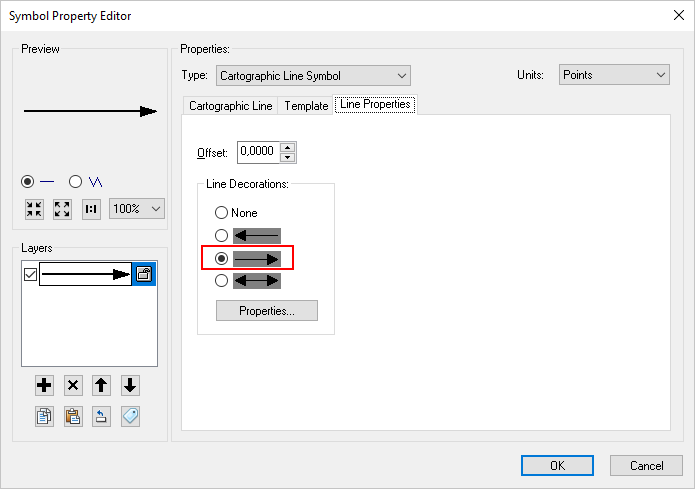Which will show you where you have problems in your data: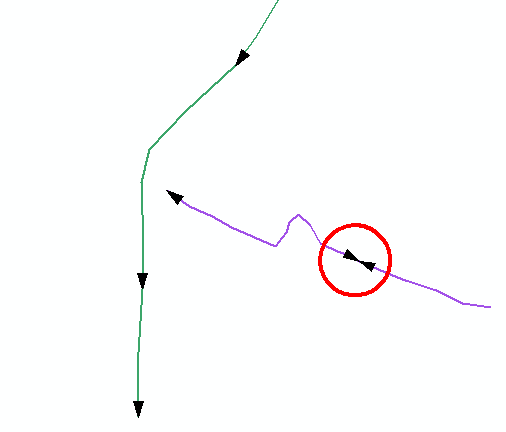You can also create end and start points in separate featureclasses form the lines using Feature Vertices to Points: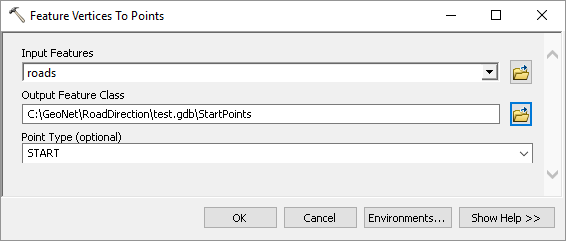And symbolize those: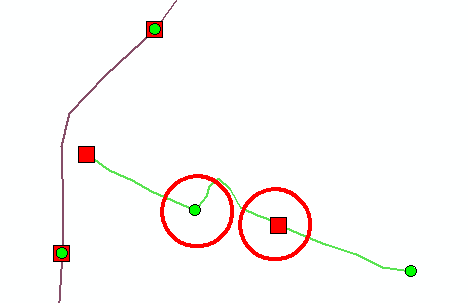You can also apply hatching (About displaying hatches—Help | ArcGIS Desktop ) to validate the M values of the road (are they continuous):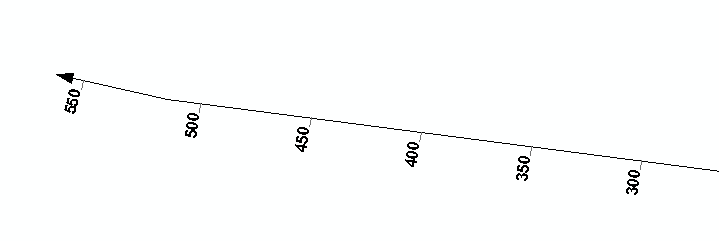Or you can analyze the start and end featureclasses with a Generate Near Table and validate where you have start points without end point (although you would need to exclude the start and end points of a route.

Or create routes to see if the routes makes sense when you apply the M values of the road segments.byMVP Esteemed Contributor

Symbology > Arrow at End.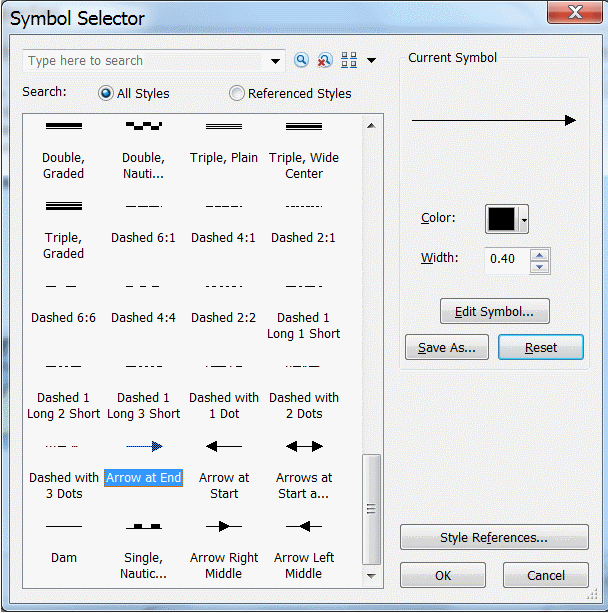Think Locationby
New Contributor II

Thank you for your answers and screenshots. Of course these solutions are good and I can apply them to my dataset.

But how to calculate (maybe in Python) distance for end point and start point of subsequent segments in every road_number (but not for first and last segments – only the middle segments) ? When the distance is more than 0 – it will be an error.byEsri Esteemed Contributor

You can script this, but this can get pretty complex. I did something in the past. Let me dig into my archive and see if I can share some code.byEsri Esteemed Contributor

See below some sample code that could be a start:

``````def main():
import arcpy

# input fc and name of field containing route name
fld_name = 'RouteName'
tolerance = 1

# sr
sr = arcpy.Describe(fc).spatialReference

# create dictionary of data
dct_routes = {}
flds = ('OID@', 'SHAPE@', fld_name)
with arcpy.da.SearchCursor(fc, flds) as curs:
for row in curs:
dct_data = {}
oid = row
polyline = row
route_name = row
if route_name in dct_routes:
dct_data = dct_routes[route_name]
dct_data[oid] = polyline
else:
dct_data[oid] = polyline
dct_routes[route_name] = dct_data

# determnine first segment of route based on minimum M value
dct_possible_errors = {}
for route_name, dct_data in sorted(dct_routes.items()):
print "dct_possible_errors", dct_possible_errors
oid = GetFirstSegmentOfRouteOnMvalue(dct_data)
print
print " - GetFirstSegmentOfRouteOnMvalue:", route_name, oid
lst_processed = [oid]
dct_start_pnts = CreateDictionaryStartOrEndPoints(dct_data, "start")
dct_end_pnts = CreateDictionaryStartOrEndPoints(dct_data, "end")
print route_name
print " - len:", len(dct_data.keys())
print " - oid:", oid

# start connecting segments
lst_todo = [o for o in dct_data.keys() if not o in lst_processed]
while len(lst_todo) != 0:
start_pnt = dct_start_pnts[oid]
end_pnt = dct_end_pnts[oid]

print " - lst_processed:", lst_processed
print " - lst_todo:", lst_todo

oid_near_end, dist_near_end, type_near_end = FindPointsNearEnd(oid, "end", dct_start_pnts, dct_end_pnts, lst_processed)
print " - FindPointsNearStartorEnd-end  :", oid_near_end, dist_near_end, type_near_end
oid_near_start, dist_near_start, type_near_start = FindPointsNearStart(oid, "start", dct_start_pnts, dct_end_pnts, lst_processed)
print " - FindPointsNearStartorEnd-start:", oid_near_start, dist_near_start, type_near_start

from_part = "end"
to_part = "not set"
if dist_near_start < tolerance:
print "   - connect to start is option..."
print "   - oid to connect to:", oid_near_start
to_part = "start"
print "ERROR possible flip line case"
if dist_near_end < tolerance:
print "   - connect to end is option..."
print "   - oid to connect to:", oid_near_end
to_part = "end"
print "    - from - to: ", from_part, " - ", to_part

if to_part == "end":
print "from_part is start, possible ERROR flip case!"
lst_todo.remove(oid_near_end)
lst_processed.append(oid_near_end)
oid = oid_near_end
print " - ERROR is end, Flip!:", oid_near_end
if route_name in dct_possible_errors:
errors = dct_possible_errors[route_name]
errors.append(oid)
dct_possible_errors[route_name] = errors
else:
dct_possible_errors[route_name] = [oid]
print " - added:", route_name, oid
elif to_part == "start":
print "to_part is end, OK"
lst_todo.remove(oid_near_start)
lst_processed.append(oid_near_start)
oid = oid_near_start
print " - OK is start        :", oid_near_start
else:
print "no candidates available in tolerance"
print " - still to do:", lst_todo
lst_todo = []

print
for route_name, errors in dct_possible_errors.items():
if len(errors) > 0:
print route_name, errors

def FindPointsNearStart(oid, start_or_end, dct_start_pnts, dct_end_pnts, lst_exclude):
if start_or_end == "start":
pnt = dct_start_pnts[oid]
else:
pnt = dct_end_pnts[oid]

dist_min = 9999
oid_min = -1
type_min = "not set"

# determine distance bewteen point and start of others
for oid_i, pnt_i in dct_start_pnts.items():
if not oid_i in lst_exclude:
dist_i = GetDistance(pnt.X, pnt.Y, pnt_i.X, pnt_i.Y)
if dist_i < dist_min:
dist_min = dist_i
oid_min = oid_i
type_min = "start"

return oid_min, dist_min, type_min

def FindPointsNearEnd(oid, start_or_end, dct_start_pnts, dct_end_pnts, lst_exclude):
if start_or_end == "start":
pnt = dct_start_pnts[oid]
else:
pnt = dct_end_pnts[oid]

dist_min = 9999
oid_min = -1
type_min = "not set"

# determine distance bewteen point and start of others
for oid_i, pnt_i in dct_end_pnts.items():
if not oid_i in lst_exclude:
dist_i = GetDistance(pnt.X, pnt.Y, pnt_i.X, pnt_i.Y)
if dist_i < dist_min:
dist_min = dist_i
oid_min = oid_i
type_min = "end"

return oid_min, dist_min, type_min

def FindPointsNearStartorEnd(oid, start_or_end, dct_start_pnts, dct_end_pnts, lst_exclude):
if start_or_end == "start":
pnt = dct_start_pnts[oid]
else:
pnt = dct_end_pnts[oid]

dist_min = 9999
oid_min = -1
type_min = "not set"
# determine distance bewteen point and start of others
for oid_i, pnt_i in dct_start_pnts.items():
if not oid_i in lst_exclude:
dist_i = GetDistance(pnt.X, pnt.Y, pnt_i.X, pnt_i.Y)
if dist_i < dist_min:
dist_min = dist_i
oid_min = oid_i
type_min = "start"

# determine distance bewteen point and start of others
for oid_i, pnt_i in dct_end_pnts.items():
if not oid_i in lst_exclude:
dist_i = GetDistance(pnt.X, pnt.Y, pnt_i.X, pnt_i.Y)
if dist_i < dist_min:
dist_min = dist_i
oid_min = oid_i
type_min = "end"

return oid_min, dist_min, type_min

def GetDistance(x1, y1, x2, y2):
import math
return math.hypot(x2-x1, y2-y1)

def CreateDictionaryStartOrEndPoints(dct_data, start_or_end):
dct_pnts = {}
for oid, polyline in dct_data.items():
if start_or_end == "start":
dct_pnts[oid] = polyline.firstPoint
else:
dct_pnts[oid] = polyline.lastPoint
return dct_pnts

def GetFirstSegmentOfRouteOnMvalue(dct_data):
m_min = 99999
oid_min = -1
for oid, polyline in dct_data.items():
for part in polyline:
for pnt in part:
m = pnt.M
if m < m_min:
m_min = m
oid_min = oid
return oid_min

if __name__ == '__main__':
main()‍‍‍‍‍‍‍‍‍‍‍‍‍‍‍‍‍‍‍‍‍‍‍‍‍‍‍‍‍‍‍‍‍‍‍‍‍‍‍‍‍‍‍‍‍‍‍‍‍‍‍‍‍‍‍‍‍‍‍‍‍‍‍‍‍‍‍‍‍‍‍‍‍‍‍‍‍‍‍‍‍‍‍‍‍‍‍‍‍‍‍‍‍‍‍‍‍‍‍‍‍‍‍‍‍‍‍‍‍‍‍‍‍‍‍‍‍‍‍‍‍‍‍‍‍‍‍‍‍‍‍‍‍‍‍‍‍‍‍‍‍‍‍‍‍‍‍‍‍‍‍‍‍‍‍‍‍‍‍‍‍‍‍‍‍‍‍‍‍‍‍‍‍‍‍‍‍‍‍‍‍‍‍‍‍‍‍‍‍‍‍‍‍‍‍‍‍‍``````

It threw as result that feature with OID 5, route "B" was in the wrong direction (although a lot of testing is still required):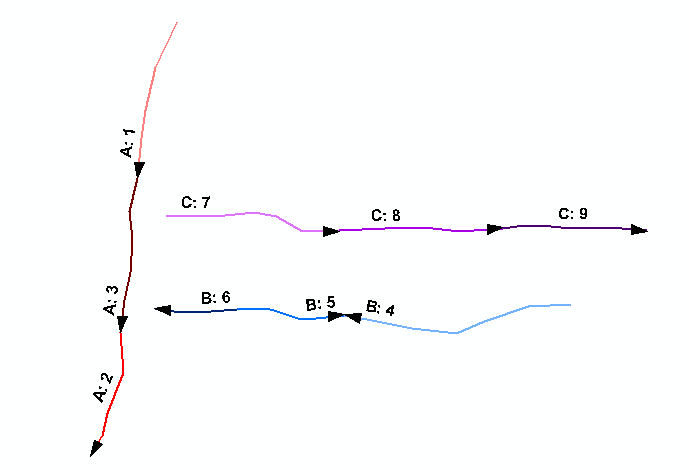Before you start to use this script, please keep the following in mind:

• This code selects the first segment (feature) based on the minimum M value
• The first feature defined the direction, if you have 10 segments in a route and the first points in a certain direction and the rest in the other, all 9 segments would be identified as "wrong"
• If you would like to define the correct direction based on the majority of the segments, you should determine if this is on number of features or combined length.
• There can be infinite exceptions that could yield undesired resultsby
New Contributor II

Thank you very much for your engagement and time you have spent on this case. I will apply and test the code. I will allow myself to contact you again in case of further questions.by
New Contributor II

I have done some test with your code on my example data. I have attached the data. You will find that:

• in my example data every segment of a given road must start with M value =0,
• the right direction is the direction of the first segment [Segment_ID] of a given road, that is the minimum value (number) in field [Segment_ID] for a given road number [RouteName]

Below screenshot of my example roads: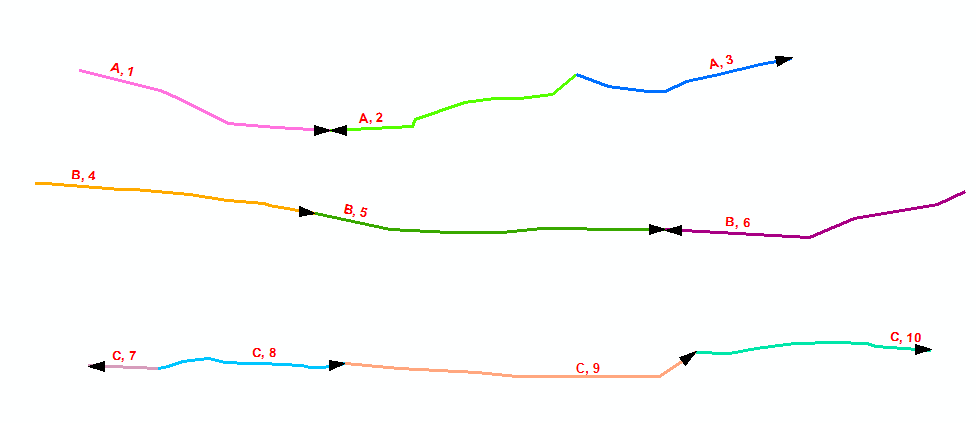The right direction for whole road indicates:

• Road A – segment 1
• Road B – segment 4
• Road C – segment 7

• Road A, Segment 2 (A, 2)
• Road B, Segment 6 (B, 6)
• Road C, Segment 8, 9, 10 (C,8; C,9; C,10)

For now, code indicates errors only at segment (A,2).by